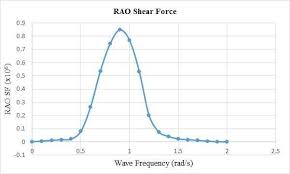## How to Calculate and Solve for Shear Force or S Waves | Rock MechanicsThe image above represents shear force or S waves.

To compute for shear force or S waves, two essential parameters are needed and these parameters are Dynamic Modulus of Rigidity (Gd) and Elasticity of Rock Mass (p).

The formula for calculating shear force or S waves:

vs = √(Gd) / p

Where:

vs = Shear Force or S Waves
Gd = Dynamic Modulus of Rigidity
p = Elasticity of Rock Mass

Let’s solve an example;
Find the shear force or s waves when the dynamic modulus of rigidity is 12 and the elasticity of rock mass is 28.

This implies that;

Gd = Dynamic Modulus of Rigidity = 12
p = Elasticity of Rock Mass = 28

vs = √(Gd) / p
vs = √(12) / 28
vs = 3.46 / 28
vs = 0.12

Therefore, the shear force or S waves is 0.12.

Calculating the Dynamic Modulus of Rigidity when the Shear Force or S Waves and the Elasticity of Rock Mass is Given.

Gd = (vs x p)2

Where:

Gd = Dynamic Modulus of Rigidity
vs = Shear Force or S Waves
p = Elasticity of Rock Mass

Let’s solve an example;
Find the dynamic modulus of rigidity when the shear force or s waves is 21 and the elasticity of rock mass is 14.

This implies that;

vs = Shear Force or S Waves = 21
p = Elasticity of Rock Mass = 14

Gd = (vs x p)2
Gd = (21 x 14)2
Gd = (294)2
Gd = 86436

Therefore, the dynamic modulus of rigidity is 86436.